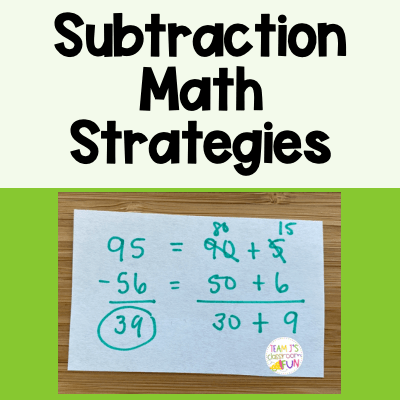Math has always been one of my favorite subjects to teach.  I think one of the main reasons why I enjoy teaching math so much is the variety of strategies that can be used to solve a math problem.  After reading this post you’ll learn four different subtraction math strategies that your students could use to help boost their understanding and math problem solving abilities.

## Subtraction Math Strategies:

Below you will see four different subtraction math strategies that your students might use.  Each student will be in a different spot with their math strategies. For example, some students need the concrete/manipulatives and place value strategy will be best for them.  Other students might be really good at breaking numbers apart and using them in different ways – then break apart or expanded might be best for them.  With math strategies there is not a one size fits all type of mentality.  Students can use a variety of strategies and will move through them at different rates when they are ready.

### Place Value Strategy:

Draw out how many there are to start with – 95  Then, take away or cross out how many are being taken away – 56.  Start by crossing out the 5 tens for 50.  Then, you have 5 ones and you can’t take away 6 from 5 so, take a ten and regroup it into 10 ones.  (Circle the ten and change it into the ten ones (dots)).  Now, you can take away the 6 ones.  Then, count what is left – 10, 20, 30, and 9 more so 39.

### Empty Number Line Strategy:

First, draw an empty number line.  Then, start at the number that you’re beginning with – 95.  95 will go on the right side of the empty number line because you are subtracting so your number will be getting smaller.  Then, 56 can be taken away a few different ways. In this example, they first took away 50 and got to 45. Then, took away 5 and got to 40 (friendly number) and one more and got to 39.  Students could also take away all 6 ones.  The 50 could also be broken down into – 20, 20, 10 – 10, 10, 10, 10, 10 – 30, 20 – 40, 10 – etc.

### Break Apart Strategy:

For break apart, leave the first number together since this is the number that you are starting with.  Then, break apart the number that you are taking away.  So, 56 would break into 50 and 6 (expanded notation).  Then, 95 – 50 = 45 and 45 – 6 = 39.

### Expanded Form Strategy:

First, change each number into expanded notation. 95 = 90 + 5 and 56 = 50 + 6.  Then, subtract the ones from the ones.  You can’t take away 6 from 5.  So, you take ten from the 90 to add to the ones.  When you take ten from the 90, the 90 turns into 80.  Then, add the ten you took to your ones 10 + 5 = 15.  Now, you can subtract.  15 – 6 = 9 and 80 – 50 = 30.  Then put the numbers back together 30 + 9 = 39.

## Subtraction Practice:

If you’re looking for some subtraction practice pages for your students to practice these strategies – I have worksheets already created for you.  Best part is – they are differentiated into 3 levels! These Subtraction Word Problem Printables are great for independent practice, homework, formative assessments, and more. Check them out here >>> Subtraction Differentiated Word Problem Worksheets.

If you are interested in learning about Addition Math Strategies, check out my blog post>>>HERE.

Previous Story
Next Story